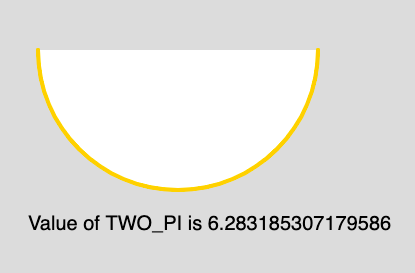p5.js | Constants | TWO_PI

The TWO_PI is a mathematical constant with the value 6.28318530717958647693. It is twice the ratio of the circumference of a circle to its diameter.

Syntax:

TWO_PI

Below program illustrates the usage of TWO_PI in p5.js:

Example:

 function setup() {            // Create Canvas of size 880*300       createCanvas(880, 300); }     function draw() {         // Set the Background Color     background(220);         // Set the stroke color     stroke(255, 204, 0);         // Set the stroke weight     strokeWeight(4);         // Draw Arc     // Use of constant TWO_PI     arc(180, 50, 280, 280, 0, TWO_PI/2);         // Unset the stroke     noStroke();         // Set the text size     textSize(20);            // Display output     text("Value of TWO_PI is "+ TWO_PI, 30, 230); }

Output:Reference: https://p5js.org/reference/#/p5/TWO_PI

My Personal Notes arrow_drop_up

Recommended Posts:

Article Tags :

Be the First to upvote.

Please write to us at contribute@geeksforgeeks.org to report any issue with the above content.